Ex 9.4

Chapter 9 Class 12 Differential Equations
Serial order wise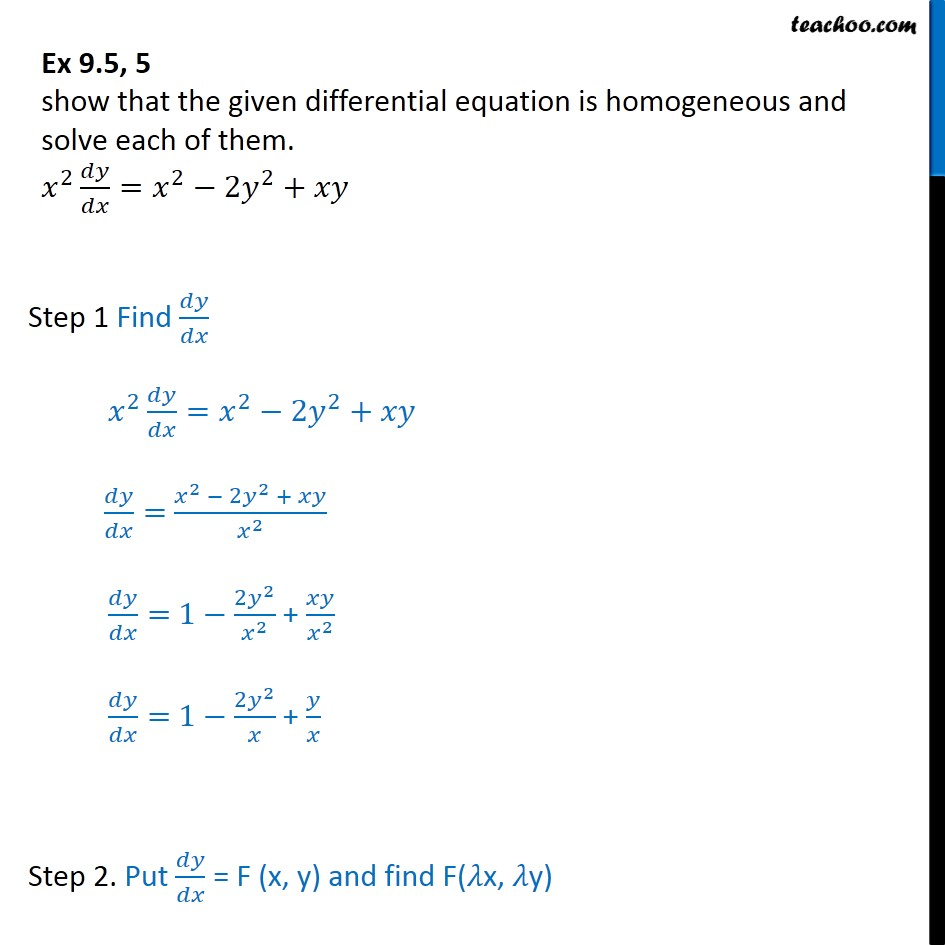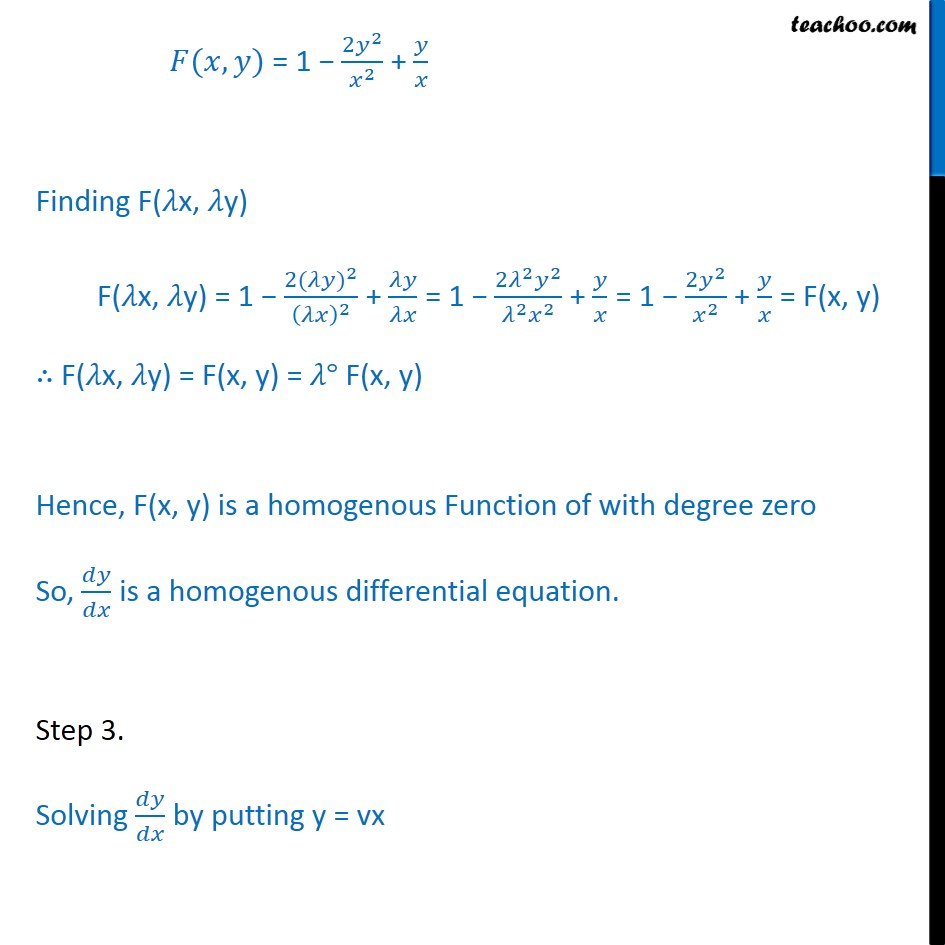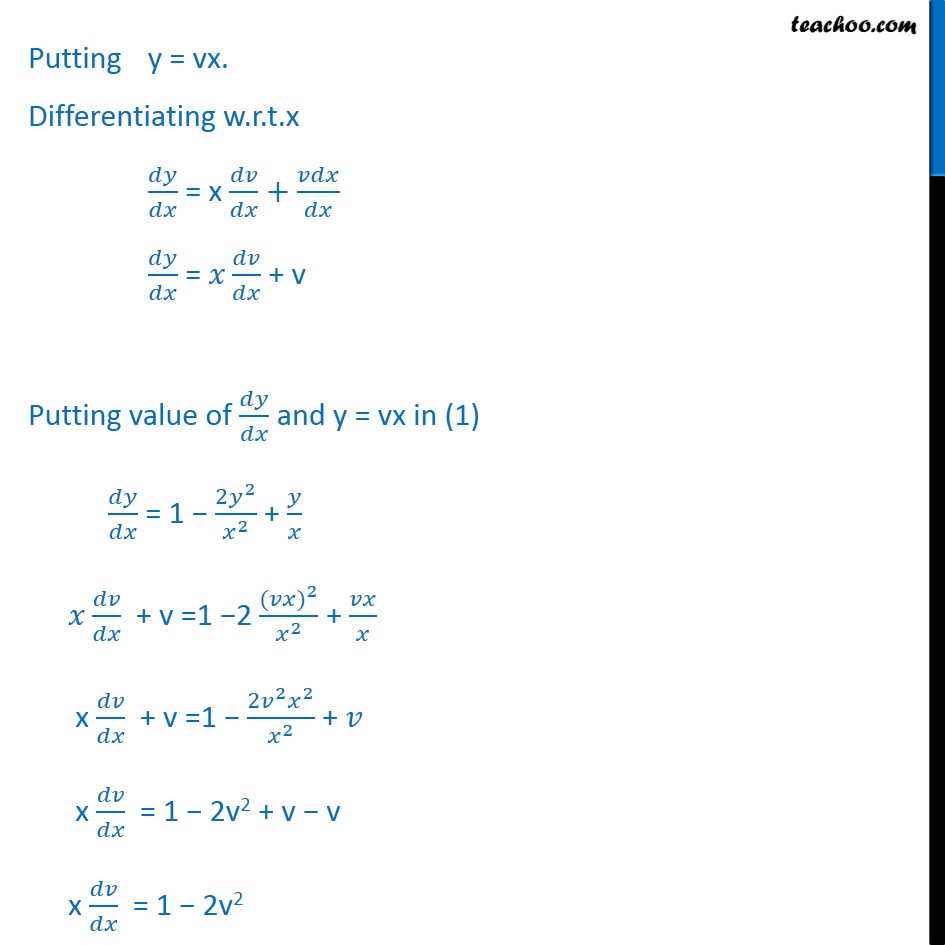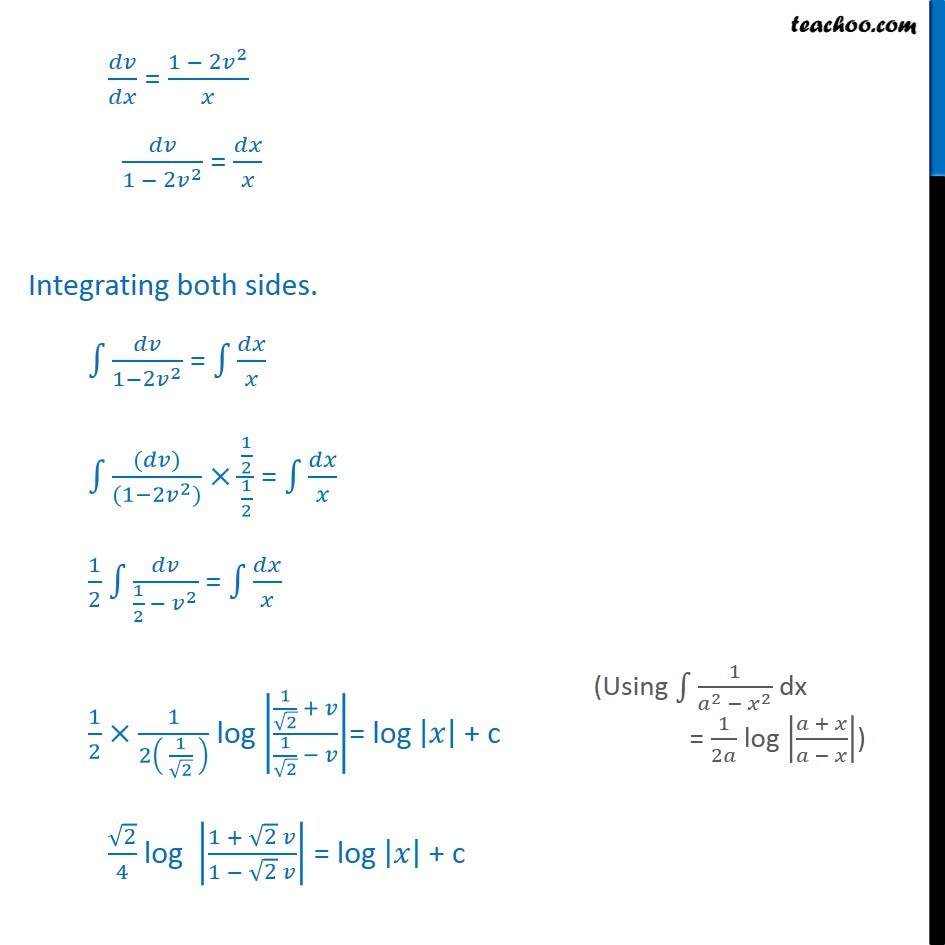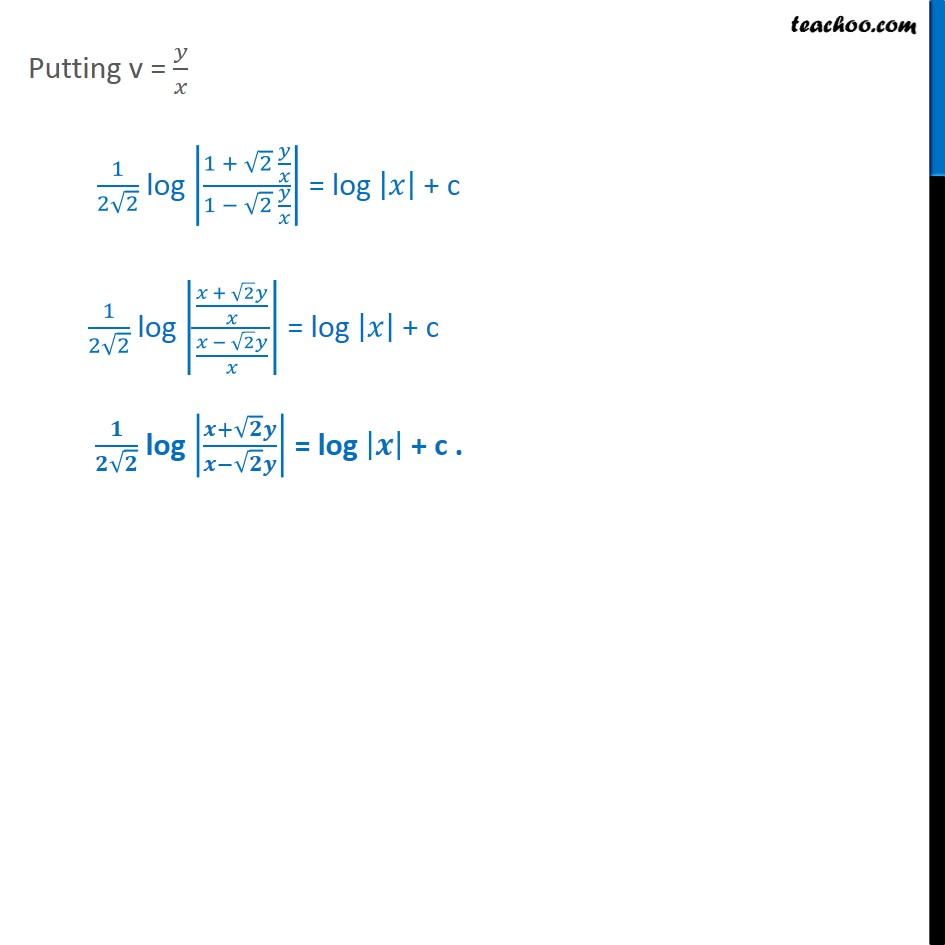Learn in your speed, with individual attention - Teachoo Maths 1-on-1 Class

### Transcript

Ex 9.4, 5 show that the given differential equation is homogeneous and solve each of them. 𝑥^2 𝑑𝑦/𝑑𝑥=𝑥^2−2𝑦^2+𝑥𝑦 Step 1 Find 𝑑𝑦/𝑑𝑥 𝑥^2 𝑑𝑦/𝑑𝑥=𝑥^2−2𝑦^2+𝑥𝑦 𝑑𝑦/𝑑𝑥= (𝑥^2 − 2𝑦^2 + 𝑥𝑦)/𝑥^2 𝑑𝑦/𝑑𝑥= 1−(2𝑦^2)/𝑥^2 + 𝑥𝑦/𝑥^2 𝑑𝑦/𝑑𝑥= 1−(2𝑦^2)/𝑥 + 𝑦/𝑥 Step 2. Put 𝑑𝑦/𝑑𝑥 = F (x, y) and find F(𝜆x, 𝜆y) 𝐹(𝑥, 𝑦) = 1 − (2𝑦^2)/𝑥^2 + 𝑦/𝑥 Finding F(𝜆x, 𝜆y) F(𝜆x, 𝜆y) = 1 − (2〖(𝜆𝑦)〗^2)/(𝜆𝑥)^2 + 𝜆𝑦/𝜆𝑥 = 1 − (2𝜆^2 𝑦^2)/(𝜆^2 𝑥^2 ) + 𝑦/𝑥 = 1 − (2𝑦^2)/𝑥^2 + 𝑦/𝑥 = F(x, y) ∴ F(𝜆x, 𝜆y) = F(x, y) = 𝜆° F(x, y) Hence, F(x, y) is a homogenous Function of with degree zero So, 𝑑𝑦/𝑑𝑥 is a homogenous differential equation. Step 3. Solving 𝑑𝑦/𝑑𝑥 by putting y = vx Putting y = vx. Differentiating w.r.t.x 𝑑𝑦/𝑑𝑥 = x 𝑑𝑣/𝑑𝑥+𝑣𝑑𝑥/𝑑𝑥 𝑑𝑦/𝑑𝑥 = 𝑥 𝑑𝑣/𝑑𝑥 + v Putting value of 𝑑𝑦/𝑑𝑥 and y = vx in (1) 𝑑𝑦/𝑑𝑥 = 1 − (2𝑦^2)/𝑥^2 + 𝑦/𝑥 𝑥 𝑑𝑣/𝑑𝑥 + v =1 −2 〖(𝑣𝑥)〗^2/𝑥^2 + 𝑣𝑥/𝑥 x 𝑑𝑣/𝑑𝑥 + v =1 − (2𝑣^2 𝑥^2)/𝑥^2 + 𝑣 x 𝑑𝑣/𝑑𝑥 = 1 − 2v2 + v − v x 𝑑𝑣/𝑑𝑥 = 1 − 2v2 𝑑𝑣/𝑑𝑥 = (1 − 2𝑣^2)/𝑥 𝑑𝑣/(1 − 2𝑣^2 ) = 𝑑𝑥/𝑥 Integrating both sides. ∫1▒𝑑𝑣/(1−2𝑣^2 ) = ∫1▒𝑑𝑥/𝑥 ∫1▒((𝑑𝑣))/((1−2𝑣^2))× (1/2)/(1/2) = ∫1▒𝑑𝑥/𝑥 1/2 ∫1▒𝑑𝑣/(1/2 − 𝑣^2 ) = ∫1▒𝑑𝑥/𝑥 1/2 ×1/2( 1/√2 ) log |(1/√2 + 𝑣)/(1/√2 − 𝑣)|= log |𝑥| + c √2/4 log |(1 + √2 𝑣)/(1 − √2 𝑣)| = log |𝑥| + c Putting v = 𝑦/𝑥 1/(2√2) log |(1 + √2 𝑦/𝑥)/(1 − √2 𝑦/𝑥)| = log |𝑥| + c 1/(2√2) log |((𝑥 + √2 𝑦)/𝑥)/((𝑥 − √2 𝑦)/𝑥)| = log |𝑥| + c 𝟏/(𝟐√𝟐) log |(𝒙+√𝟐 𝒚)/(𝒙−√𝟐 𝒚)| = log |𝒙| + c .